# A MACD Implementation in Python From Scratch

Disclaimer: This post is for entertainment/educational purposes only. The content of this post is not meant to provide investment advice or to help with investment decision making.

QUICK-NAV:
MACD
Implementation
Full Code

Moving Average Convergence Divergence (MACD [MAK-DEE]) is a trading algorithm that uses the price momentum of a security to define buying and selling opportunities. The algorithm works by monitoring the convergence/divergence of two different moving averages (MAs) of the security’s price (one long MA and one short MA) and uses a moving average of this convergence/divergence measurement (known as the signal line) to signal buying and selling opportunities. Typically, the long MA uses 26 periods, the short MA uses 12 periods and the signal line uses 9 periods where the MAs are computed as Exponential Moving Averages (EMAs) which give more weight to recent data points. Such a process is known as MACD(12, 26, 9). Although these numbers typically work well there’s nothing absolute about them, any of the EMAs can be modified to use a different number of periods to signal different trading opportunities.

When looking for implementations of this algorithm to gain insight on its inner workings I found almost no from-scratch implementations in Python. Most of the implementations in Python use libraries that already have the algorithm implemented so the logic boils down to, essentially, a few function calls. In this post, I will discuss the MACD crossover indicator and develop a Python implementation from scratch. This implementation will be wrapped in a Python class making it easy to use in other projects.

## MACD

### Exponential Moving Averages (EMAs)

As mentioned above, MACD is a trend-following momentum indicator that uses the short-term momentum of a security’s price to signal trading opportunities. The momentum is determined by calculating the exponential moving average (EMA) for a long-term horizon and a short-term horizon, most commonly 26-periods and 12-periods, respectively. The EMA is defined as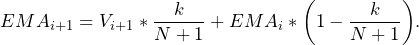Here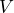is the value of the time series,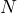is the number of periods, and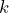is a smoothing factor which is usually set to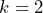. In each of these terms (and in any following equations) the subscriptis the time step with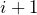being the current time step andbeing the previous time step. As seen above, the EMA depends on the previous value of the EMA. This raises the question of how to start the calculations. What is typically done, and what was done here, is using a different calculation of the moving average, known as the Simple Moving Average (SMA), of the last N time periods. The SMA is the typical average we’re all familiar with, defined as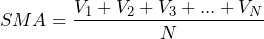with the values defined in the same way as above. Below is a graph of the opening stock prices of 3M (MMM) from 07/06/2019 – 07/06/2020 with the long-term EMA (red) and the short-term EMA (green) overlayed on the price (blue). It can be seen here that the EMAs cross during upswings and downswings, this will be taken advantage of with MACD, after adding a little more complexity.

### MACD

Now that we have a definition of the EMA and a plan on how to start the recursive calculation, MACD can be defined. This post will be focusing on the common MACD(12, 26, 9) indicator meaning the long-term EMA is a 26-period EMA and the short-term EMA is a 12-period EMA. With this, we define the MACD as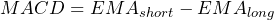Note here that there is one exception to the subscript rule. In this case, long and short are not time steps but are used to indicate the long and short moving averages. As seen in the MACD equation,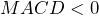when the short-term EMA drops below the long-term EMA and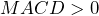when the short-term EMA rises above the long-term EMA. When plotting the MACD line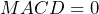is known as the baseline. The more the value is above or below the baseline the larger the divergence (distance between) the long- and short-term EMAs.

### The Signal Line

The algorithm uses one more moving average to determine when to buy or sell the security known as the signal line. This moving average is different in that, rather than being calculated from the security’s price, it is calculated from previous and current values of the MACD line. Plotted below are the MACD and the 9-period (day) EMA of the MACD which correspond to the 3M data shown above.

The signal line and the MACD line are used to signal trading opportunities. The MACD line crossing above the signal line is an indication to purchase the security, while a cross below the signal lines indicates a selling opportunity. Below is another graphic for 3M. This time both of the graphs above are included, and buy/sell lines in green/red are added to the stock price chart. These lines were determined by the MACD line crossing above or below the signal line which can be seen in the second graph.

If the actions are taken above for 3M between 7/6/2019 and 7/6/2020, the total profit would be 20.74%. The trades determined by the MACD crossover indicator for this data can be seen below. Note that the initial sell indicator is ignored since we would have nothing to sell at that time in this case.

Below is the difference between subsequent buys and sells. The profit is calculated from this table as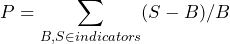where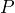is the profit,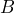is the purchasing price, and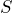is the selling price.

### A Second Example

The indicator doesn’t always offer favorable results. For example, trading the security Alliance Data Systems Corporation (ADS) over the same time period results in a loss of 16.51%. The trades and trade data are shown in the graph and tables below.

## Python Implementation

### Gathering Data

In a previous post (Medium link), I discussed the implementation of an API wrapper that pulls historical daily, weekly, and monthly stock data from Yahoo Finance. I won’t be repeating that discussion here and have decided not to include the source code due to the length of this post. To learn more about how the data was gathered feel free to read through the posts linked above. If you want to use your own data the only requirement is that the data has headers and a Date column (case sensitive) and that you pass in the column name for the stock price data. When using your own data you will also need to modify the __get_data() method defined below.

### The DailyMACD Class

The DailyMACD class is used to run the algorithm and process the results. The implementation only deals with daily data but could be modified to use data streams with different frequencies. The only thing that I can think of that would need to be changed is the processing of the results and Date column as days (mostly display issues). The implementation should still work the same no matter the frequency of the data provided. Below is a skeleton of the class as well as the libraries we will be using.

from yahoo_api import YahooAPI
import datetime
import matplotlib.pyplot as plt
from dateutil.relativedelta import relativedelta
import numpy as np
import pandas as pd

class DailyMACD(object):
def __init__(self, ticker, years, short_prd, long_prd, signal_long_length,
signal_short_length=0, tolerance=0.002, end_date=None,
column="Open"):
pass

def __get_data(self):
pass

def __sma(self, N, price_hist):
pass

def __ema(self, N, curr_price, past_ema):
pass

def get_macd(self):
return self.macd

def get_signal(self):
return self.signal

def get_long_ema(self):
return self.long_ema

def get_short_ema(self):
return self.short_ema

pass

def purchase_prices(self):
pass

def sell_prices(self):
pass

def profit(self):
pass

def get_data(self):
pass

def volatility(self):
pass

def ticker_symbol(self):
pass

pass

def view(self):
pass

def run(self):
pass

#### Setup

To get started there are a few things that need to be set up for the algorithm to run. First in foremost, in the constructor, we need to store the data being provided by the user including the ticker symbol, the length of the EMAs, and the column containing the price data. The class also takes an end_date which can be used to specify the last day for which we want to get data. The total date range is defined as (end_date – years) through end_date. The constructor also creates the Yahoo Finanace API object which will be used to retrieve data. After everything is initialized, the data is fetched.

def __init__(self, ticker, years, short_prd, long_prd, signal_long_length,
signal_short_length=0, end_date=None,
column="Open"):
self.ticker = ticker # the ticker symbol
self.years = years # number of years in the past to get data
self.long = long_prd # long EMA
self.short = short_prd # short EMA
self.signal_long_length = signal_long_length # signal line EMA
self.purchase_prices = []
self.sell_prices = []
self.end_date = datetime.datetime.today() if (end_date is None) else end_date
self.data = None
self.signal_short_length = signal_short_length # for future post
self.column = column # column with price data

self.api = YahooAPI()
self.__get_data()

The private __get_data() function is used to fetch the data for the stock ticker symbol within the date range defined above. This function uses the YahooAPI object’s get_ticker_data() function which returns a Pandas dataframe containing the data for the ticker symbol within the date range. Then the data is sorted (although it already should be) and split into two subsets. The first subset is used to get the ball rolling for the short-term EMA, long-term EMA, and signal line EMA. The second subset is the price data that will be considered for trading opportunities.

def __get_data(self):
start_date = self.end_date - relativedelta(years=self.years)
try:
self.data = self.api.get_ticker_data(self.ticker, start_date, self.end_date)
except:
return

self.data = self.data.sort_values("Date")
self.ema_data  = self.data.loc[:(self.long + self.signal_long_length)-1]
self.data = self.data.loc[(self.long + self.signal_long_length):]

self.data_dates = pd.to_datetime(self.data.Date, format="%Y-%m-%d").tolist()
self.ema_dates = pd.to_datetime(self.ema_data.Date, format="%Y-%m-%d").tolist()
self.long_sma_data = self.ema_data.loc[:self.long-1][self.column]
self.short_sma_data = self.ema_data.loc[:self.short-1][self.column]

#### Running the Algorithm

Now that we have the data required to determine buy and sell triggers we can run the MACD crossover algorithm on the data. First, we need to define two private functions that are used to calculate the SMA and EMA.

def __sma(self, N, price_hist):
return sum(price_hist) / N

def __ema(self, N, curr_price, past_ema):
# "Smoothing Factor"
k = 2 / (N + 1)
ema = (curr_price * k) + (past_ema * (1-k))
return ema

Now we’re ready to get to the heart of the algorithm to determine trading opportunities. This is taken care of in the run() function of the DailyMACD class.

def run(self):
# use first <long/short> # of points to start the EMA
# since it depends on previous EMA
long_sma_value = self.__sma(self.long, self.long_sma_data)
short_sma_value = self.__sma(self.short, self.short_sma_data)
self.long_ema = [long_sma_value]
self.short_ema = [short_sma_value]

# need to remove these values at the end
# 'use up' the remainder of the data for the EMAs
for index, v in self.ema_data[self.long:].iterrows():
self.long_ema.append(self.__ema(self.long, v[self.column], self.long_ema[-1]))
for index, v in self.ema_data[self.short:].iterrows():
self.short_ema.append(self.__ema(self.short, v[self.column], self.short_ema[-1]))

# calculate the EMA values for the long/short lines for the
# actual data under consideration (non-EMA data)
for index, value in self.data.iterrows():
self.long_ema.append(self.__ema(self.long, value[self.column], self.long_ema[-1]))
self.short_ema.append(self.__ema(self.short, value[self.column], self.short_ema[-1]))
# remove the first few values from the short EMA list
# to catch up with the start of the long EMA list
self.short_ema = self.short_ema[(self.long - self.short):]

# create numpy arrays to easily difference EMAs
self.long_ema = np.asarray(self.long_ema)
self.short_ema = np.asarray(self.short_ema)
self.macd = self.short_ema - self.long_ema

# use the first N values to start signal line EMA calc
signal_line_sma = self.__sma(self.signal_long_length, self.macd[-self.signal_long_length:])
self.long_signal = [signal_line_sma]
# calculate the signal line for the actual (non-EMA) data
for m in self.macd[self.signal_long_length+1:]:
self.long_signal.append(self.__ema(self.signal_long_length, m, self.long_signal[-1]))
# remove first entry in signal since it was only used to start calc
self.long_signal = self.long_signal[1:]
# remove the first few values of macd/short/long
# emas to catch up with signal/data
self.macd = self.macd[self.signal_long_length+1:]
self.long_ema = self.long_ema[self.signal_long_length+1:]
self.short_ema = self.short_ema[self.signal_long_length+1:]

# get difference of MACD and signal to find crossings
self.long_signal = np.asarray(self.long_signal)
self.diffs = self.macd - self.long_signal

self.sell_lines = []
for i in range(1, len(self.diffs)):
# previous MACD was < signal and current is greater so  buy
if self.diffs[i-1] < 0 and self.diffs[i] > 0:
# previous MACD was > signal and current is less so  sell
if self.diffs[i-1] > 0 and self.diffs[i] < 0:
self.sell_lines.append(i)

The above logic is the most difficult part of the implementation to follow. Essentially the EMA calculations for the short-term, long-term, and signal line need to get started by computing the SMA values for each line. The SMA is calculated with the firstvalues (being the number of values for the EMA in question). The data for these initial calculations are taken from the EMA dataset retrieved in the __get_data() function. This data is then ignored since we won’t have all of the necessary information in these first values to detect trading opportunities. So throughout the run() function, we slice the data a few times to remove this data from the EMA lines.

Note that, although we remove the EMA data from the EMA lines and the overall dataset, the EMAs for the short- and long-term lines use all of this data so the EMAs used when starting the algorithm are actually the EMA values at the start of the price data. For MACD(12, 26, 9) 35 data points are removed (number of long-term periods + signal line periods). The short-term EMA is started with the first 12 of these data points then calculated for the next 14 data points (to total 26). Then the long-term EMA uses the first 26 data points meaning the short-term and long-term EMAs have both used the same 26 data points and are the same length. The difference between these two EMAs is then taken and the last 9 values in this line (the MACD line) are used to start the signal line EMA calculation. Afterward, all of the data is sliced so that it gets ‘caught up’ with the price data we’ll be using to run the algorithm.

At the end of this procedure, there will be a list of buy and sell indices into the price data dataframe. Some intuition is needed when using these lists. For example, we can’t sell before we’ve bought (neglecting short-selling) even if the algorithm tells us to and we are able to sell the security at the final price in the dataset even if the algorithm doesn’t tell us to. With that in mind, the following function is used to calculate the buying prices, selling prices, and total profits when taking recommendations from the MACD indicator.

def get_buy_sell_profits(self):
position = 0 # have we bought yet?
self.purchase_prices = []
self.sell_prices = []
for i in range(len(self.data)):
if position == 0:   # must have sold before buying
position = 1
self.purchase_prices.append(self.data.iloc[i][self.column])
if i in self.sell_lines:
if position > 0:    # must purchase before selling
position = 0
self.sell_prices.append(self.data.iloc[i][self.column])
if len(self.purchase_prices) > len(self.sell_prices):
# purchased at the end, consider accumulated profit/loss
self.sell_prices.append(self.data.iloc[-1][self.column])
self.purchase_prices = np.asarray(self.purchase_prices)
self.sell_prices = np.asarray(self.sell_prices)
# as percentage/100
self.profit = np.sum((self.sell_prices - self.purchase_prices)/self.purchase_prices)

return self.purchase_prices, self.sell_prices, self.profit

The function above iterates all of the price data for the security and determines if we are buying or selling at that price depending on the output of the run() function and whether or not we are able to buy or sell. Afterwards, these trade prices are used to calculate the profits made from the algorithm as discussed above in the MACD overview. All of this data is returned to the user when calling this function.

#### Viewing the Results

Now that we have buying and selling points and prices and what the total profit was from using this strategy, it would be nice to be able to view and analyze this data. The most useful method for doing so is the view() function. This function generates and shows the graphs included in the MACD section of this post.

def view(self):
fig, (ax1, ax2) = plt.subplots(2, 1, gridspec_kw={'height_ratios': [4, 1]})
fig.suptitle(self.ticker.upper() + " - MACD (" + str(self.short) + ", " \
+ str(self.long) + ", " + str(self.signal_long_length) + ")")

ax2.set_title("MACD vs Signal")
ax2.set_ylabel("EMA")
ax2.set_xlabel("Date")
# plot the macd and signal lines on the bottom
ax2.plot(self.data_dates, self.macd, color="green", label="MACD")
ax2.plot(self.data_dates, self.long_signal, color="red",
label=(str(self.signal_long_length) +"-Period EMA"))
ax2.legend()
ax2.grid(True) # looks a little nicer

ax1.set_title("Price Data")
ax1.set_xlabel("Date")
ax1.set_ylabel("Price")
# plot the long and short EMA lines
ax1.plot(self.data_dates, self.long_ema, color="tomato", label="long ema")
ax1.plot(self.data_dates, self.short_ema, color="olivedrab", label="short ema")
# plot the price data
ax1.plot(self.data_dates, self.data[self.column], color="blue", label="price")
# display the buying and selling points
for line in self.sell_lines:
ax1.axvline(self.data_dates[line], color="red")
ax1.axvline(self.data_dates[line], color="green")
ax1.legend()
ax1.grid(True) # looks a little nicer

plt.show() # display the graph

The DailyMACD class also offers some methods to retrieve the data generated by running the algorithm. The function names are pretty self-explanatory so no further discussion will be given.

def get_buy_sell_dates(self):
sell_dates = []
for i in self.sell_lines:
sell_dates.append(self.data_dates[i])

def get_macd(self):
return self.macd

def get_signal(self):
return self.signal

def get_long_ema(self):
return self.long_ema

def get_short_ema(self):
return self.short_ema

def purchase_prices(self):
return self.purchase_prices

def sell_prices(self):
return self.sell_prices

def profit(self):
return self.profit

def get_data(self):
return self.data

def volatility(self):
return np.std(self.data[self.column])

def ticker_symbol(self):
return self.ticker.upper()

### A Sample Run

Below is what a run of MACD(12, 26, 9) might look like when using this class in separate Python logic.

from DailyMACD import DailyMACD

if __name__ == "__main__":
# define MACD object
macd = DailyMACD('intc', 1, 12, 26, 9)
# run the algorithm
macd.run()
# calculate the profits and buy/sell prices
# get the buy sell dates
print(sell)  # prices sold at
print(profit) # total profit
macd.view() # view graphs

## Conclusion

In this post, I’ve covered the basics of MACD and provided an implementation in Python. Although I focused on the MACD(12, 26, 9) crossover indicator these values can be changed to find different trading opportunities (some perhaps more profitable and others less profitable). One thing to note is that the entire process doesn’t need to be run every time we want to make a trading decision (buy, sell, hold). The short- and long-term EMAs, the signal line, and the MACD can all be retrieved from the DailyMACD class. This data can then be stored and used to make subsequent decisions via these calculations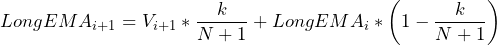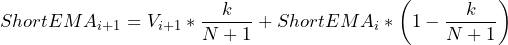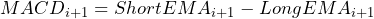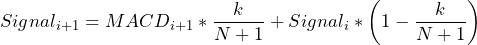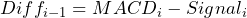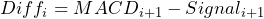where V is the value of the security, k is the smoothing factor, and N is the number of periods corresponding to the individual EMAs (e.g. either 12, 26, or 9 in the example in this post). Then the values for Diff can be compared (as they are at the end of the run() function) to provide a buy, sell, or hold signal.

### Full Code

from yahoo_api import YahooAPI
import datetime
import matplotlib.pyplot as plt
from dateutil.relativedelta import relativedelta
import numpy as np
import pandas as pd

class DailyMACD(object):
def __init__(self, ticker, years, short_prd, long_prd, signal_long_length,
signal_short_length=0, end_date=None,
column="Open"):
self.ticker = ticker
self.years = years
self.long = long_prd
self.short = short_prd
self.signal_long_length = signal_long_length
self.purchase_prices = []
self.sell_prices = []
self.end_date = datetime.datetime.today() if (end_date is None) else end_date
self.data = None
self.signal_short_length = signal_short_length
self.column = column

self.api = YahooAPI()
self.__get_data()

def __get_data(self):
start_date = self.end_date - relativedelta(years=self.years)
try:
self.data = self.api.get_ticker_data(self.ticker, start_date, self.end_date)
except:
return

self.data = self.data.sort_values("Date")
self.ema_data  = self.data.loc[:(self.long + self.signal_long_length)-1]
self.data = self.data.loc[(self.long + self.signal_long_length):]

self.data_dates = pd.to_datetime(self.data.Date, format="%Y-%m-%d").tolist()
self.ema_dates = pd.to_datetime(self.ema_data.Date, format="%Y-%m-%d").tolist()
self.long_sma_data = self.ema_data.loc[:self.long-1][self.column]
self.short_sma_data = self.ema_data.loc[:self.short-1][self.column]

def __sma(self, N, price_hist):
return sum(price_hist) / N

def __ema(self, N, curr_price, past_ema):
# "Smoothing Factor"
k = 2 / (N + 1)
# ema = (curr_price - past_ema) * k + past_ema
ema = (curr_price * k) + (past_ema * (1-k))
return ema

sell_dates = []
for i in self.sell_lines:
sell_dates.append(self.data_dates[i])

def purchase_prices(self):
return self.purchase_prices

def sell_prices(self):
return self.sell_prices

def profit(self):
return self.profit

def get_data(self):
return self.data

def volatility(self):
return np.std(self.data[self.column])

def ticker_symbol(self):
return self.ticker.upper()

position = 0
self.purchase_prices = []
self.sell_prices = []
for i in range(len(self.data)):
if position == 0:   # must have sold before buying
position = 1
self.purchase_prices.append(self.data.iloc[i][self.column])
if i in self.sell_lines:
if position > 0:    # must purchase before selling
position = 0
self.sell_prices.append(self.data.iloc[i][self.column])
if len(self.purchase_prices) > len(self.sell_prices):
# purchased at the end, consider accumulated profit/loss
self.sell_prices.append(self.data.iloc[-1][self.column])
self.purchase_prices = np.asarray(self.purchase_prices)
self.sell_prices = np.asarray(self.sell_prices)

# as percentage/100
self.profit = np.sum((self.sell_prices - self.purchase_prices)/self.purchase_prices)

return self.purchase_prices, self.sell_prices, self.profit

def view(self):
fig, (ax1, ax2) = plt.subplots(2, 1, gridspec_kw={'height_ratios': [4, 1]})
fig.suptitle(self.ticker.upper() + " - MACD (" + str(self.short) + ", " \
+ str(self.long) + ", " + str(self.signal_long_length) + ")")

ax2.set_title("MACD vs Signal")
ax2.set_ylabel("EMA")
ax2.set_xlabel("Date")
ax2.plot(self.data_dates, self.macd, color="green", label="MACD")
ax2.plot(self.data_dates, self.long_signal, color="red",
label=(str(self.signal_long_length) +"-Period EMA"))
if self.signal_short_length > 0:
ax2.plot(self.dates, self.short_signal, color="orange",
label=(str(self.signal_short_length) +"-Period EMA"))
ax2.legend()
ax2.grid(True)

ax1.set_title("Price Data")
ax1.set_xlabel("Date")
ax1.set_ylabel("Price")
ax1.plot(self.data_dates, self.long_ema, color="tomato", label="long ema")
ax1.plot(self.data_dates, self.short_ema, color="olivedrab", label="short ema")
ax1.plot(self.data_dates, self.data[self.column], color="blue", label="price")
for line in self.sell_lines:
ax1.axvline(self.data_dates[line], color="red")
ax1.axvline(self.data_dates[line], color="green")
ax1.legend()
ax1.grid(True)

plt.show()

def run(self):
# use first <long/short> # of points to start the EMA since it depends on previous EMA
long_sma_value = self.__sma(self.long, self.long_sma_data)
short_sma_value = self.__sma(self.short, self.short_sma_data)
self.long_ema = [long_sma_value]
self.short_ema = [short_sma_value]

# need to remove these values at the end
for index, v in self.ema_data[self.long:].iterrows():
self.long_ema.append(self.__ema(self.long, v[self.column], self.long_ema[-1]))
for index, v in self.ema_data[self.short:].iterrows():
self.short_ema.append(self.__ema(self.short, v[self.column], self.short_ema[-1]))

# calculate the remainded of the EMA values for the long/short lines
for index, value in self.data.iterrows():
self.long_ema.append(self.__ema(self.long, value[self.column], self.long_ema[-1]))
self.short_ema.append(self.__ema(self.short, value[self.column], self.short_ema[-1]))
# remove the first few values from the short EMA list to catch up with the
# start of the long EMA list
self.short_ema = self.short_ema[(self.long - self.short):]

self.long_ema = np.asarray(self.long_ema)
self.short_ema = np.asarray(self.short_ema)
self.macd = self.short_ema - self.long_ema

# use the first N values to start signal line EMA calc
signal_line_sma = self.__sma(self.signal_long_length, self.macd[-self.signal_long_length:])
self.long_signal = [signal_line_sma]
for m in self.macd[self.signal_long_length+1:]:
self.long_signal.append(self.__ema(self.signal_long_length, m, self.long_signal[-1]))
# remove first entry in signal since it was only used to start calc
self.long_signal = self.long_signal[1:]
# remove the first few values of macd/short/long emas to catch up with signal/data
self.macd = self.macd[self.signal_long_length+1:]
self.long_ema = self.long_ema[self.signal_long_length+1:]
self.short_ema = self.short_ema[self.signal_long_length+1:]

self.long_signal = np.asarray(self.long_signal)
self.diffs = self.macd - self.long_signal

self.sell_lines.append(i)